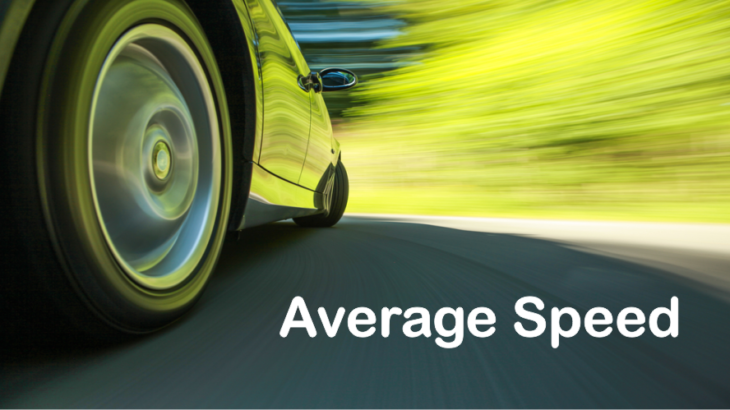# Average Speed: Time Speed and Distance

Average Speed: Time Speed and Distance
5 (100%) 7 votes

Average speed of an object is defined as the ratio of the total distance covered to the total time taken. Mainly there are two different types of questions based on the concept of average speed.

The first type is when a body travels the same distance at different speeds i.e. the distance is constant. And the second is when a body travels at different speeds, each speed for equal duration i.e. the time is constant.

### Average speed when the distance is constant

A body is traveling constant distance with two different speed say X and Y respectively then the average speed for its entire journey is the harmonic mean of X and Y.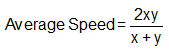Derivation

Refer to the figure given below. Let us assume that a person moves from A to B with a speed of x and from B to A with a speed of y.  Also, let the distance between AB be  D.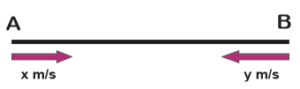The total distance traveled by him = 2D.

Time taken by him to travel from A to B = D/x  and from B to A = D/y.

Therefore, the total time taken by him during his entire journey = D/x+D/y

Now,  the average speed= Total distance/total time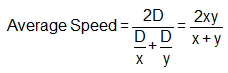Let us understand the application of the formula with help of few examples

Example: If Aakash goes from Raipur to Bilaspur at a speed of 30 km per hour and comes back at a speed of 70 km per hour then what is his average speed during the entire journey?

Explanation

Here we can see that Akash is traveling from Raipur to Bilaspur and back to Raipur. In this case, we can say that he is traveling the same distance which two different speed that is 30 km per hour and 70 km per hour.

Therefore, the average speed would be the harmonic mean of the two speed or we can say that:

The average speed= 2x30x70/(30+70)= 42 km per hour.

Let’s take one more example

Example: A person goes around an equilateral triangle shaped field at a speed for X, Y and Z kilometer per hour on the first, second and the third side. Find his average speed during the journey

Explanation

Now, this question is not different than the previous question. The only difference is that here the person is traveling 3 equal distance each at different speeds.

In this is also, the average speed would be the harmonic mean of all the three speeds given. Let the measure of each side of the triangle be D kilometer.

The person traveled the distance from A to B with X kilometer per hour, B to C with Y kilometer per hour and C to A with Z kilometer per hour.

Time taken by him to travel from A to B = D/x.

Similarly, the time taken to travel from B to C and C to A is D/Y and D/Z  respectively.

So, the total time taken by him = D/x+D/y+D/z, and the total distance traveled is 3D

His average speed=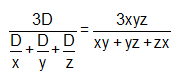Example: A bird flying 400 km covers the first 100 KM @ 100 km per hour, the second 100 KM at 200 km per hour, third 100 KM @ 300 km per hour and the last 100 KM @ 400 km per hour. Determine the average speed of the bird in the entire journey.

Explanation

Total time taken= 100/100 +   100/200 + 100/300+100/400=25/12 hours

Therefore, average speed =400/(25/12)=192 kmph.

I hope the above examples clear the concept of average speed in case of same distance traveled at different speeds.

### Average speed when the time is constant

When a body moves with different speed but for an equal duration in each time then the average speed of the entire journey is the Arithmetic mean of all the speeds.

Example:  Rahul travels with the speed of 20 km per hour for the first 2 hours. For the next two hours, he travels with a speed of 30 km per hour. Find the average speed of his entire journey.

Explanation

Total distance travelled = 20×2+30×2=100 kilometers

Total time taken =2+2 = 4 hours

Therefore, the average speed=100/4=25 kmph.

## Time Speed and Distance